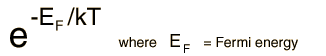# The Fermi-Dirac Distribution

The Fermi-Dirac distribution applies to fermions, particles with half-integer spin which must obey the Pauli exclusion principle. Each type of distribution function has a normalization term multiplying the exponential in the denominator which may be temperature dependent. For the Fermi-Dirac case, that term is usually written:The significance of the Fermi energy is most clearly seen by setting T=0. At absolute zero, the probability is =1 for energies less than the Fermi energy and zero for energies greater than the Fermi energy. We picture all the levels up to the Fermi energy as filled, but no particle has a greater energy. This is entirely consistent with the Pauli exclusion principle where each quantum state can have one but only one particle.Distribution functions Numerical example
 Fermi level in solids Fermi level in band theory of solids
Index

Applied statistics concepts

 HyperPhysics***** Quantum Physics R Nave
Go Back

# Fermi-Dirac DetailsThe Fermi-Dirac distribution.
Index

Applied statistics concepts

 HyperPhysics***** Quantum Physics R Nave
Go Back